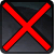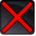# [flurry_ranged_dual_wield]Conditions

## Related effects, buffs and debuffs

• [0s] [not displayed]Effect #1

 Slot: Debuff Duration: 0s Tick rate: does not tick # occurrences: 0
• On Apply

Only when the following conditions are met:

• <
• If a random chance of 50% is met
AND
• If TARGET is dual wielding, with weapon mode 3
>

Perform the following actions:

• Play appearance epp . flurry . flurry_ranged_primary
• Weapon Damage
- Is Special Ability = (bool) false
- Ignore Dual Wield Modifier = (bool) false
- Unknown (609) = (bool) false
- Flurry Blows Min = (int) 0
- Flurry Blows Max = (int) 0
- Level Cap = (int) 0
- Threat Percent = (float) 0
- Standard Health Percent Max = (float) 0
- Standard Health Percent Min = (float) 0
- Amount Modifier Fixed Max = (float) 0
- Amount Modifier Fixed Min = (float) 0
- Amount Modifier Percent = (float) 0
- Coefficient = (float) 0
- Health Steal Percentage = (float) 0
- Slots = Int(2)
• Weapon Damage
- Is Special Ability = (bool) false
- Ignore Dual Wield Modifier = (bool) false
- Unknown (609) = (bool) false
- Flurry Blows Min = (int) 0
- Flurry Blows Max = (int) 0
- Level Cap = (int) 0
- Threat Percent = (float) 0
- Standard Health Percent Max = (float) 0
- Standard Health Percent Min = (float) 0
- Amount Modifier Fixed Max = (float) 0
- Amount Modifier Fixed Min = (float) 0
- Amount Modifier Percent = (float) 0
- Coefficient = (float) 0
- Health Steal Percentage = (float) 0
- Slots = Int(4)
• On Apply

Only when the following conditions are met:

• If previous subeffect did not meet all conditions

Perform the following actions:

• Play appearance epp . flurry . flurry_ranged_primary
• Weapon Damage
- Is Special Ability = (bool) false
- Ignore Dual Wield Modifier = (bool) false
- Unknown (609) = (bool) false
- Flurry Blows Min = (int) 0
- Flurry Blows Max = (int) 0
- Level Cap = (int) 0
- Threat Percent = (float) 0
- Standard Health Percent Max = (float) 0
- Standard Health Percent Min = (float) 0
- Amount Modifier Fixed Max = (float) 0
- Amount Modifier Fixed Min = (float) 0
- Amount Modifier Percent = (float) 0
- Coefficient = (float) 0
- Health Steal Percentage = (float) 0
- Slots = Int(2)